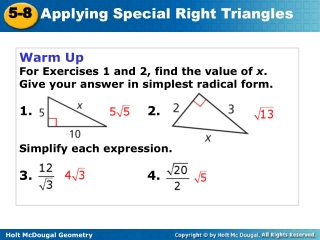DownloadDownload PresentationWarm Up For Exercises 1 and 2, find the value of x . Give your answer in simplest radical form.

# Warm Up For Exercises 1 and 2, find the value of x . Give your answer in simplest radical form.

Télécharger la présentation## Warm Up For Exercises 1 and 2, find the value of x . Give your answer in simplest radical form.

- - - - - - - - - - - - - - - - - - - - - - - - - - - E N D - - - - - - - - - - - - - - - - - - - - - - - - - - -
##### Presentation Transcript

1. Warm Up For Exercises 1 and 2, find the value of x. Give your answer in simplest radical form. 1.2. Simplify each expression. 3.4.

2. Standard U5S9 I can justify and apply properties of 45°-45°-90° and 30°- 60°- 90° triangles.

3. Example 1A: Finding Side Lengths in a 45°- 45º- 90º Triangle Find the value of x. Give your answer in simplest radical form.

4. Example 1B: Finding Side Lengths in a 45º- 45º- 90º Triangle Find the value of x. Give your answer in simplest radical form.

5. Check It Out! Example 1a Find the value of x. Give your answer in simplest radical form.

6. Check It Out! Example 1b Find the value of x. Give your answer in simplest radical form.

7. A 30°-60°-90° triangle is another special right triangle. You can use an equilateral triangle to find a relationship between its side lengths.

8. Example 3A: Finding Side Lengths in a 30º-60º-90º Triangle Find the values of x and y. Give your answers in simplest radical form.

9. Example 3B: Finding Side Lengths in a 30º-60º-90º Triangle Find the values of x and y. Give your answers in simplest radical form.

10. Check It Out! Example 3a Find the values of x and y. Give your answers in simplest radical form.

11. Check It Out! Example 3b Find the values of x and y. Give your answers in simplest radical form.

12. Check It Out! Example 3c Find the values of x and y. Give your answers in simplest radical form.

13. Check It Out! Example 3d Find the values of x and y. Give your answers in simplest radical form.

14. Homework: p. 372 #1-3, 5-7 textbook